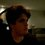# Who is right? (Complex fraction problem)

Hello everyone, that's my first discussion (open-ended post), so if there are any improvements to be done, then please don't hesitate in informing about it.

Getting to the point, there's an equation which I've seen in some wiki from Brilliant, and it's really bothering me because I can't understand it at all. I tried to solve it myself, and I got a completely different result. I also entered it in some math computing websites (such as WolframAlpha), but they also gave me completely different results from the equation I've seen around here. I'm going to post both ways (the way I've seen, Computation A, and my way, Computation B) so someone might be able to point which of both are right (or explain why none are right).

## Computation A

$\LARGE \frac{ \frac{ x+1 }{ x-1 } + \frac{ x-1 }{ x+1} }{ \frac{ x+1 }{ x-1 } + \frac{ x-1 }{ x+1} } = \frac{ \frac{(x+1)²+(x-1)²}{(x-1)(x+1)} }{ \frac{(x+1)²-(x-1)²}{(x-1)(x+1)} } = \frac{ \frac{x²+2x+1+x²-2x+1}{x²-1} }{ \frac{x²+2x+1-(x²-2x+1)}{x²-1} } = \frac{2x²+2}{x²-1} \times \frac{x²-1}{4x} = \frac{x²+1}{2x}$

## $\LARGE x \neq 0, x \neq \pm1$

I'm still in high-school level on math, but in either way I wasn't able to follow that math. Not completely, but I'll keep an ordered list on how I interpreted the steps in case anyone can clarify anything to me. Each number from the list refers to its respective step in the Computation A equation.

1. Okay, that was given.
2. That's where I lost track. I mean, he probably just multiplied both fractions from the numerator and denominator by their respective LCMs, but here's what I find strange there: if the numerator and denominator in the largest fraction are the same, then why did they turned into different products when simplified? And if they're the same, then why isn't the fracion't quotient 1? Anyway, from that result we know that $x \neq \pm 1$.
3. Further simplification. I get that.
4. Ok... A bunch of steps were skipped from the third to the fourth step, but if you simplify the third step yourself you'll get that result (I hope so). From this step we can infer that $x \neq 0$ as well.
5. Simplification from previous step, but it makes sense to me.

Now here's my way of solving it when considering that $\frac{a}{a} = 1$

## Computation B

$\LARGE \frac{\frac{x+1}{x-1}+\frac{x-1}{x+1}}{\frac{x+1}{x-1}+\frac{x-1}{x+1}}$

$\LARGE a = \frac{x+1}{x-1}+\frac{x-1}{x+1}$

## $\LARGE \therefore \frac{\frac{x+1}{x-1}+\frac{x-1}{x+1}}{\frac{x+1}{x-1}+\frac{x-1}{x+1}} = \frac{a}{a} = 1$

And by the way, I don't trust my own calculations and methods, so I threw that problem into some online math-computing programs. There are the links:Note by Daniel Maia
5 years ago

This discussion board is a place to discuss our Daily Challenges and the math and science related to those challenges. Explanations are more than just a solution — they should explain the steps and thinking strategies that you used to obtain the solution. Comments should further the discussion of math and science.

When posting on Brilliant:

• Use the emojis to react to an explanation, whether you're congratulating a job well done , or just really confused .
• Ask specific questions about the challenge or the steps in somebody's explanation. Well-posed questions can add a lot to the discussion, but posting "I don't understand!" doesn't help anyone.
• Try to contribute something new to the discussion, whether it is an extension, generalization or other idea related to the challenge.

MarkdownAppears as
*italics* or _italics_ italics
**bold** or __bold__ bold
- bulleted- list
• bulleted
• list
1. numbered2. list
1. numbered
2. list
Note: you must add a full line of space before and after lists for them to show up correctly
paragraph 1paragraph 2

paragraph 1

paragraph 2

[example link](https://brilliant.org)example link
> This is a quote
This is a quote
    # I indented these lines
# 4 spaces, and now they show
# up as a code block.

print "hello world"
# I indented these lines
# 4 spaces, and now they show
# up as a code block.

print "hello world"
MathAppears as
Remember to wrap math in $$ ... $$ or $ ... $ to ensure proper formatting.
2 \times 3 $2 \times 3$
2^{34} $2^{34}$
a_{i-1} $a_{i-1}$
\frac{2}{3} $\frac{2}{3}$
\sqrt{2} $\sqrt{2}$
\sum_{i=1}^3 $\sum_{i=1}^3$
\sin \theta $\sin \theta$
\boxed{123} $\boxed{123}$

Sort by:

The answer is indeed 1. After taking L.C.M in the denominator, you did subtraction instead of addition.

That's what I thought. Actually the "Computation A" I got from a wiki page from Brilliant itself, but I thought it was really weird. But thanks for the input anyway!

- 5 years ago

Seems to be a typo. It'll be better if someone corrects it to prevent more people from being confused.

Do you remember which wiki page?

Yep. It was the fractions wiki, but don't worry, I'll change the plus sign from the denominator to a minus sign, so it should get corrected.

- 5 years ago